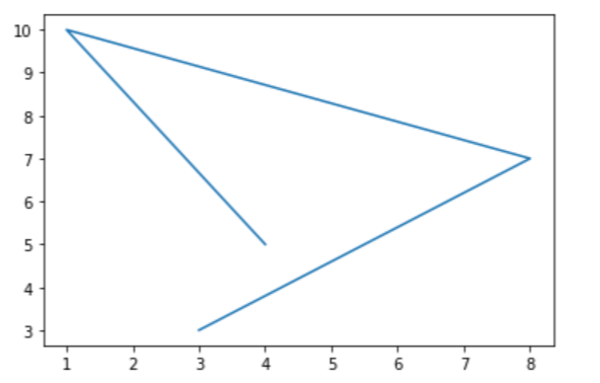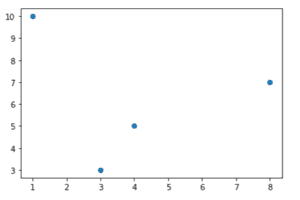Trusted answers to developer questions
Trusted Answers to Developer Questions

Related Tags

python
scalar
data
visualization
communitycreator

# What is matplotlib.pyplot.plot() in Python?

Eman Kashif

Grokking Modern System Design Interview for Engineers & Managers

Ace your System Design Interview and take your career to the next level. Learn to handle the design of applications like Netflix, Quora, Facebook, Uber, and many more in a 45-min interview. Learn the RESHADED framework for architecting web-scale applications by determining requirements, constraints, and assumptions before diving into a step-by-step design process.

Data visualization is a significant aspect of data science. It is used to present data in a sensible manner for statistical or comparative analysis.

matplotlib is a Python library that provides a multitude of functions to visualize and plot data.

The matplotlib.pyplot.plot() function is used to plot two scalar values against each other as lines or markers.

### Syntax

plot([x], y, [fmt], *, data=None, **kwargs)


### Parameters

• x,y: Can be arrays or scalars. They correspond to the x-axis and y-axis values. The x-axis values are optional and the default value of x is range(len(y)).

• fmt: A string value that specifies the formatting of the lines. (Optional)

• data: A object that contains labeled data. (Optional)

### Return value

matplotlib.pyplot.plot() returns a list of Line2D objects that represent the data.

### Code

The code below plots a line that joins multiple points.

#import librariesimport matplotlib.pyplot as pltimport numpy as np#initialize x-axis and y-axis valuesx = np.array([1, 2, 3, 4])y = np.array([1, 4, 9, 16])#plot a lineplt.plot(x, y)plt.show()To plot points without a line, the relevant format string is passed as parameter.

#import librariesimport matplotlib.pyplot as pltimport numpy as np#initialize y-axis values with default x-axis valuesy = np.array([5, 10, 7, 3])#plot points onlyplt.plot(x, y, 'o')plt.show()RELATED TAGS

python
scalar
data
visualization
communitycreator

CONTRIBUTOR

Eman Kashif

Grokking Modern System Design Interview for Engineers & Managers

Ace your System Design Interview and take your career to the next level. Learn to handle the design of applications like Netflix, Quora, Facebook, Uber, and many more in a 45-min interview. Learn the RESHADED framework for architecting web-scale applications by determining requirements, constraints, and assumptions before diving into a step-by-step design process.

Keep Exploring

Learn in-demand tech skills in half the time